### Example 64.1 One-Compartment Model with Pharmacokinetic Data

A popular application of nonlinear mixed models is in the field of pharmacokinetics, which studies how a drug disperses through a living individual. This example considers the theophylline data from Pinheiro and Bates (1995). Serum concentrations of the drug theophylline are measured in 12 subjects over a 25-hour period after oral administration. The data are as follows.

data theoph;
input subject time conc dose wt;
datalines;
1  0.00  0.74 4.02 79.6
1  0.25  2.84 4.02 79.6
1  0.57  6.57 4.02 79.6
1  1.12 10.50 4.02 79.6
1  2.02  9.66 4.02 79.6
1  3.82  8.58 4.02 79.6
1  5.10  8.36 4.02 79.6
1  7.03  7.47 4.02 79.6

... more lines ...

12 24.15  1.17 5.30 60.5
;


Pinheiro and Bates (1995) consider the following first-order compartment model for these data: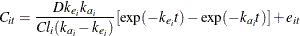where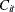is the observed concentration of the ith subject at time t, D is the dose of theophylline,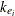is the elimination rate constant for subject i,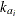is the absorption rate constant for subject i,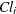is the clearance for subject i, and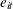are normal errors. To allow for random variability between subjects, they assume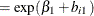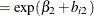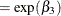where thes denote fixed-effects parameters and the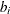s denote random-effects parameters with an unknown covariance matrix.

The PROC NLMIXED statements to fit this model are as follows:

proc nlmixed data=theoph;
parms beta1=-3.22 beta2=0.47 beta3=-2.45
s2b1 =0.03  cb12 =0    s2b2 =0.4 s2=0.5;
cl   = exp(beta1 + b1);
ka   = exp(beta2 + b2);
ke   = exp(beta3);
pred = dose*ke*ka*(exp(-ke*time)-exp(-ka*time))/cl/(ka-ke);
model conc ~ normal(pred,s2);
random b1 b2 ~ normal([0,0],[s2b1,cb12,s2b2]) subject=subject;
run;


The PARMS statement specifies starting values for the threes and four variance-covariance parameters. The clearance and rate constants are defined using SAS programming statements, and the conditional model for the data is defined to be normal with mean pred and variance s2. The two random effects are b1 and b2, and their joint distribution is defined in the RANDOM statement. Brackets are used in defining their mean vector (two zeros) and the lower triangle of their variance-covariance matrix (a general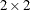matrix). The SUBJECT= variable is subject.

The results from this analysis are as follows.

Output 64.1.1: Model Specification for One-Compartment Model

The NLMIXED Procedure

Specifications
Data Set WORK.THEOPH
Dependent Variable conc
Distribution for Dependent Variable Normal
Random Effects b1 b2
Distribution for Random Effects Normal
Subject Variable subject
Optimization Technique Dual Quasi-Newton

The Specifications table lists the setup of the model (Output 64.1.1). The Dimensions table indicates that there are 132 observations, 12 subjects, and 7 parameters. PROC NLMIXED selects 5 quadrature points for each random effect, producing a total grid of 25 points over which quadrature is performed (Output 64.1.2).

Output 64.1.2: Dimensions Table for One-Compartment Model

Dimensions
Observations Used 132
Observations Not Used 0
Total Observations 132
Subjects 12
Max Obs Per Subject 11
Parameters 7

The Parameters table lists the 7 parameters, their starting values, and the initial evaluation of the negative log likelihood using adaptive Gaussian quadrature (Output 64.1.3). The Iteration History table indicates that 10 steps are required for the dual quasi-Newton algorithm to achieve convergence.

Output 64.1.3: Starting Values and Iteration History

Parameters
beta1 beta2 beta3 s2b1 cb12 s2b2 s2 NegLogLike
-3.22 0.47 -2.45 0.03 0 0.4 0.5 177.789945

Iteration History
Iter   Calls NegLogLike Diff MaxGrad Slope
1   5 177.776248 0.013697 2.873367 -63.0744
2   8 177.7643 0.011948 1.698144 -4.75239
3   10 177.757264 0.007036 1.297439 -1.97311
4   12 177.755688 0.001576 1.441408 -0.49772
5   14 177.7467 0.008988 1.132279 -0.8223
6   17 177.746401 0.000299 0.831293 -0.00244
7   19 177.746318 0.000083 0.724198 -0.00789
8   21 177.74574 0.000578 0.180018 -0.00583
9   23 177.745736 3.88E-6 0.017958 -8.25E-6
10   25 177.745736 3.222E-8 0.000143 -6.51E-8

 NOTE: GCONV convergence criterion satisfied.

Output 64.1.4: Fit Statistics for One-Compartment Model

Fit Statistics
-2 Log Likelihood 355.5
AIC (smaller is better) 369.5
AICC (smaller is better) 370.4
BIC (smaller is better) 372.9

The Fit Statistics table lists the final optimized values of the log-likelihood function and information criteria in the smaller is better form (Output 64.1.4).

Output 64.1.5: Parameter Estimates for One-Compartment Model

Parameter Estimates
Parameter Estimate Standard Error DF t Value Pr > |t| Alpha Lower Upper Gradient
beta1 -3.2268 0.05950 10 -54.23 <.0001 0.05 -3.3594 -3.0942 -0.00009
beta2 0.4806 0.1989 10 2.42 0.0363 0.05 0.03745 0.9238 3.645E-7
beta3 -2.4592 0.05126 10 -47.97 <.0001 0.05 -2.5734 -2.3449 0.000039
s2b1 0.02803 0.01221 10 2.30 0.0445 0.05 0.000833 0.05523 -0.00014
cb12 -0.00127 0.03404 10 -0.04 0.9710 0.05 -0.07712 0.07458 -0.00007
s2b2 0.4331 0.2005 10 2.16 0.0560 0.05 -0.01353 0.8798 -6.98E-6
s2 0.5016 0.06837 10 7.34 <.0001 0.05 0.3493 0.6540 6.133E-6

The Parameter Estimates table contains the maximum likelihood estimates of the parameters (Output 64.1.5). Both s2b1 and s2b2 are marginally significant, indicating between-subject variability in the clearances and absorption rate constants, respectively. There does not appear to be a significant covariance between them, as seen by the estimate of cb12.

The estimates of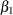,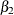, and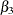are close to the adaptive quadrature estimates listed in Table 3 of Pinheiro and Bates (1995). However, Pinheiro and Bates use a Cholesky-root parameterization for the random-effects variance matrix and a logarithmic parameterization for the residual variance. The PROC NLMIXED statements using their parameterization are as follows, and results are similar.

proc nlmixed data=theoph;
parms ll1=-1.5 l2=0 ll3=-0.1 beta1=-3 beta2=0.5 beta3=-2.5 ls2=-0.7;
s2   = exp(ls2);
l1   = exp(ll1);
l3   = exp(ll3);
s2b1 = l1*l1*s2;
cb12 = l2*l1*s2;
s2b2 = (l2*l2 + l3*l3)*s2;
cl   = exp(beta1 + b1);
ka   = exp(beta2 + b2);
ke   = exp(beta3);
pred = dose*ke*ka*(exp(-ke*time)-exp(-ka*time))/cl/(ka-ke);
model conc   ~ normal(pred,s2);
random b1 b2 ~ normal([0,0],[s2b1,cb12,s2b2]) subject=subject;
run;